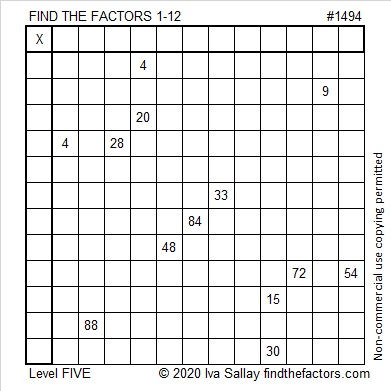# 1494 and Level 5

Contents

### Today’s Puzzle:

Write the numbers from 1 to 12 in both the first column and the top row so that the given clues are the products of those factors. Be sure to use logic to find the factors! Guessing and checking will only frustrate you.### Factors of 1494:

• 1494 is a composite number.
• Prime factorization: 1494 = 2 × 3 × 3 × 83, which can be written 1494 = 2 × 3² × 83
• 1494 has at least one exponent greater than 1 in its prime factorization so √1494 can be simplified. Taking the factor pair from the factor pair table below with the largest square number factor, we get √1494 = (√9)(√166) = 3√166
• The exponents in the prime factorization are 1, 2, and 1. Adding one to each exponent and multiplying we get (1 + 1)(2 + 1)(1 + 1) = 2 × 3 × 2 = 12. Therefore 1494 has exactly 12 factors.
• The factors of 1494 are outlined with their factor pair partners in the graphic below.### Other Facts about the Number 1494:

1494 is not the sum of or the difference of two squares, but it is still a part of three Pythagorean triples because of these three ways it can be factored:
1494 = 2(747)(1),
1494 = 2(249)(3), and
1494 = 2(83)(9).
And because for whole numbers where a > b, 2(a)(b), a² – b², a² + b² will be a Pythagorean triple.

This site uses Akismet to reduce spam. Learn how your comment data is processed.